## Thursday, 7 August 2014

### chapter-23 Interesting Probability Questions

The subject of probability gives rise to some very interesting questions, which we believe everyone should be exposed to, even if to a minor extent. In this section, we’ll do precisely that. The examples will clearly show you the depth of this subject.
 Example: 23
 A drunk mathematician called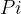$Pi$ is performing a random walk along the number line as follows. His original position is$x = 0$. He takes a step forward or a step backward with equal probability. Thus, for example, if he is at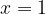$x = 1$, he can go to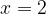$x = 2$ or$x = 0$ with equal probability. His home is at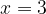$x = 3$, while there is a pit at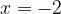$\,x = - 2$. What is the probability that he’ll reach home before falling into the pit?Solution: 18
Assume the required probability to be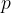$p$. Thus,$p$ denotes the probability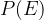$P(E)$ where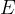$E$ :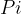$Pi$ reaches home before the pit
Now, let us draw the probability tree that will make$E$ happen:What should the events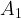${A_1}$ and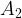${A_2}$ be and what should be their probabilities? Once$Pi$ has reached$x = 1$, the situation is as follows:${A_1}$ should therefore be the event that$Pi$ reaches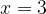$x = 3$ before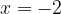$x = - 2$. Now comes the crucial insight. Compare Fig-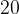$20$ with Fig-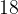$18$. The situations have been symmetrically reversed. In Fig -$18$$p$ was the probability that$Pi$ reaches$x = 3$ before$x = - 2$, that is,$Pi$ travels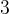$3$ units in one direction before travelling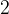$2$ in the other. In Fig-$20$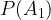$P({A_1})$ is the probability that$Pi$ travels$2$ units in one direction before travelling$3$ in the other, so we simply have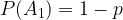$P({A_1}) = 1 - p$
Make sure you understand this argument carefully.
Coming to${A_2}$ ,$Pi$ has reached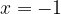$x = - 1$, he must return to$x = 0$, because he must not reach$x = - 2$. Once he is at$x = 0$, he comes back to the starting configuration, which means that now his probability of reaching$x = 3$ before$x = - 2$ is again$p$.
So let us complete the probability tree of Fig-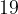$19$ now:Thus (pay attention to how we write this!),$p\,\, = \,\,\dfrac{1}{2}\,\, \times \,\,\mathop {(1 - p)}\limits_{\scriptstyle{\rm{Reaching}}\;\;x = 3\;\hfill\atop \scriptstyle{\rm{ from}}\;x = 1\hfill} \,\, + \,\,\dfrac{1}{2}\,\,\, \times \,\,\,\dfrac{1}{2}\,\, \times \mathop p\limits_{\scriptstyle{\rm{Back}}\;{\rm{to}}\hfill\atop \scriptstyle\,\,\,\,x = 0\hfill}$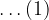$\ldots(1)$
This relation is a form of recursion. In the definition (or expression) of$p$, we are using$p$ again. Thus, recursion is, in plain words, the process a ‘procedure’ goes through when one of the steps of the ‘procedure’ involves rerunning the procedure. In this example, the ‘procedure’ of calculating$p$ involved the step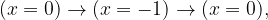$(x = 0) \to (x = - 1) \to (x = 0),$ at which point we have to rerun the ‘procedure’ of calculating$p$.
From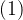$(1)$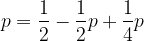$p = \dfrac{1}{2} - \dfrac{1}{2}p + \dfrac{1}{4}p$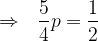$\Rightarrow\,\,\,\, \dfrac{5}{4}p = \dfrac{1}{2}$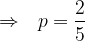$\Rightarrow\,\,\,\, p = \dfrac{2}{5}$
Thus, there is a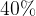$40\%$ chance that$Pi$ will return home safely!
Recursions can also take the following form: suppose we have to calculate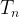${T_n}$, the${n^{th}}$term of some sequence, and suppose we are able to express${T_n}$ as some function of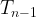${T_{n - 1}}$, the previous term: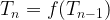${T_n} = f({T_{n - 1}})$
This is also a recursion relation, for now we can use this relation repeatedly to descend, that is, to reach some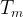${T_m}$ that is calculable: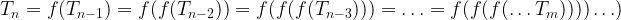${T_n} = f({T_{n - 1}}) = f(f({T_{n - 2}})) = f(f(f({T_{n - 3}}))) = \ldots = f(f(f(\ldots {T_m}))))\ldots )$
For example,${T_m}$ could be the first term of the sequence. Thus, here we are rerunning the ‘procedure’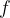$f$.